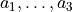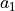# sfepy.homogenization.utils module¶

sfepy.homogenization.utils.build_op_pi(var, ir, ic)[source]

Pi_i^{rs} = y_s delta_{ir} for r = ir, s = ic.

sfepy.homogenization.utils.coor_to_sym(ir, ic, dim)[source]
sfepy.homogenization.utils.create_pis(problem, var_name)[source]

Pi_i^{rs} = y_s delta_{ir}, ul{y} in Y coordinates.

sfepy.homogenization.utils.create_scalar_pis(problem, var_name)[source]

Pi^k = y_k, ul{y} in Y coordinates.

sfepy.homogenization.utils.define_box_regions(dim, lbn, rtf=None, eps=0.001, kind='facet')[source]

Define sides and corner regions for a box aligned with coordinate axes.

Parameters
dimint

Space dimension

lbntuple

Left bottom near point coordinates if rtf is not None. If rtf is None, lbn are the (positive) distances from the origin.

rtftuple

Right top far point coordinates.

epsfloat

A parameter, that should be smaller than the smallest mesh node distance.

kindbool, optional

The region kind.

Returns
regionsdict

The box regions.

sfepy.homogenization.utils.get_box_volume(dim, lbn, rtf=None)[source]

Volume of a box aligned with coordinate axes.

Parameters:

dimint

Space dimension

lbntuple

Left bottom near point coordinates if rtf is not None. If rtf is None, lbn are the (positive) distances from the origin.

rtftuple

Right top far point coordinates.

Returns:

volumefloat

The box volume.

sfepy.homogenization.utils.get_lattice_volume(axes)[source]

Volume of a periodic cell in a rectangular 3D (or 2D) lattice.

Parameters
axesarray

The array with the periodic cell axesas rows.

Returns
volumefloat

The periodic cell volume. In 2Dwith zeros as the third components of vectors,.

Get volume of a given region using integration defined by a given field. Both the region and the field have to be defined in problem.

sfepy.homogenization.utils.integrate_in_time(coef, ts, scheme='forward')[source]

Forward difference or trapezoidal rule. ‘ts’ can be anything with ‘times’ attribute.

sfepy.homogenization.utils.interp_conv_mat(mat, ts, tdiff)[source]
sfepy.homogenization.utils.iter_nonsym(dim)[source]
sfepy.homogenization.utils.iter_sym(dim)[source]
sfepy.homogenization.utils.rm_multi(s)[source]
sfepy.homogenization.utils.set_nonlin_states(variables, nl_state, problem)[source]

Setup reference state for nonlinear homogenization

Parameters
variablesdict

All problem variables

nl_statereference state
problemproblem description